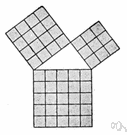theorem

(redirected from Theorum)
Also found in: Thesaurus, Medical, Legal, Encyclopedia.

the·o·rem

(thē′ər-əm, thîr′əm)
n.
1. An idea that has been demonstrated as true or is assumed to be so demonstrable.
2. Mathematics A proposition that has been or is to be proved on the basis of explicit assumptions.

[Late Latin theōrēma, from Greek, from theōrein, to look at, from theōros, spectator; see theory.]

theorem

(ˈθɪərəm)
n
(Logic) maths logic a statement or formula that can be deduced from the axioms of a formal system by means of its rules of inference
[C16: from Late Latin theōrēma, from Greek: something to be viewed, from theōrein to view]
theorematic, theoremic, theorematical adj

the•o•rem

(ˈθi ər əm, ˈθɪər əm)

n.
1. Math. a theoretical proposition, statement, or formula embodying something to be proved from other propositions or formulas.
2. a rule or law, esp. one expressed by an equation or formula.
3. Logic. a proposition that can be deduced from the premises or assumptions of a system.
4. an idea, method, or statement generally accepted as true or worthwhile without proof.
[1545–55; < Late Latin theōrēma < Greek theṓrēma spectacle, object of contemplation, theorem =theōrē-, variant s. of theōreîn to observe, derivative of theōrós person sent to consult an oracle, spectator + -ma resultative n. suffix]
the`o•re•mat′ic (-əˈmæt ɪk) adj.

the·o·rem

(thē′ər-əm, thîr′əm)
A mathematical statement whose truth can be proved on the basis of a given set of axioms or assumptions.
ThesaurusAntonymsRelated WordsSynonymsLegend:
 Noun 1theorem - a proposition deducible from basic postulatesbinomial theorem - a theorem giving the expansion of a binomial raised to a given powerproposition - (logic) a statement that affirms or denies something and is either true or false 2 theorem - an idea accepted as a demonstrable truthidea, thought - the content of cognition; the main thing you are thinking about; "it was not a good idea"; "the thought never entered my mind"Bayes' theorem - (statistics) a theorem describing how the conditional probability of a set of possible causes for a given observed event can be computed from knowledge of the probability of each cause and the conditional probability of the outcome of each cause

theorem

noun He postulated a theorem and proved it.

theorem

noun
A broad and basic rule or truth:
Translations
نَظَرِيَّه
teorém
læresætning
elméleti tétel
setning
teorema
teorēma
teoréma
davateorem

theorem

[ˈθɪərəm] N (Math) →

[ˈθiːərəm] n

theorem

nSatz m (also Math), → Theorem nt (geh, spec)

theorem

[ˈθɪərəm] n (Math) →

theorem

(ˈθiərəm) noun
especially in mathematics, something that has been or must be proved to be true by careful reasoning. a geometrical theorem.
References in periodicals archive ?
Edward Bragiel, for Mr Davies, told the judge that, as a 13-yearold, he struck on a novel geometrical method of producing drawings of animal heads after being set the task of "demonstrating an understanding of Blaise Pascal's Hexagrammum Mysticum Theorum" as a punishment by a French teacher.
And those skills have served me well in my life - which is more than I can say for Shakespeare or Pythagoras' theorum.
Analysis of the mutation frequency data at the TK locus for binary mixtures of BaP and PhIP by the median effect equation and the combination index theorum (CI), interaction factor (IF), or independent action (IA).

Site: Follow: Share:
Open / Close